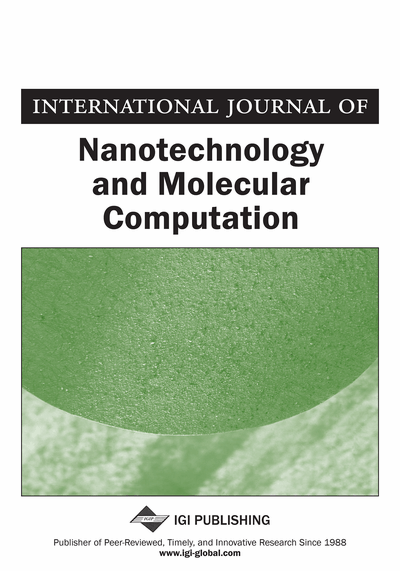# An Analytically Tractable Model of Large Network: Dynamics and Reliability

S. Vakulenko (Saint Petersburg State University of Technology and Design and Institute of Mechanical Engineering Problems, Russia) and M. Zimin (Saint Petersburg State University of Technology and Design, Russia)
Copyright: © 2010 |Pages: 12
DOI: 10.4018/jnmc.2010010101
OnDemand PDF Download:
Available
\$37.50
No Current Special Offers

## Abstract

This paper considers specially organized networks of large size. They can serve as models of computer communication systems, economical systems, neural and genetic networks. The topology of this network is simple and the analysis of the network behaviour is an analytically tractable task, while computer simulations are difficult. The authors show that such networks generate any structurally stable attractors in particular chaotic and periodic. They can simulate all Turing machines, that is, perform any computations. In noisy cases, the reliability of such network is exponentially high as a function of network size and has a maximum for an optimal network size.
Article Preview
Top

## 1. Introduction

In last decades, a large attention has been given to problems of global organization, stability and evolution of complex networks such as neural and gene networks, economical systems, Internet (see, for example, Albert & Barabasi, 2002; Lesne, 2006; Reinitz & Sharp, 1995; Sornette, 2003). In this paper we consider dynamical network models having the form

(1.1) where t = 0, 1, 2, ...,, ξi (t) are random real valued functions of discrete time t. Here only ξi are random, h,Kij are parameters that we adjust in order to control the network behaviour. We set initial conditions ui (0) ≡ vi . The function σ is an increasing function satisfying limz→−∞ σ(z) =0, limz→∞ σ(z) = 1. Typical examples of such functions are as follows:

The corresponding continuous time analogue of this system is given by, (1.2)where λi, ri > 0. Systems are basic for neural and gene network theory (Hopfield, 1982; Glass & Kauffman, 1973; Reinitz & Sharp, 1995; Lesne, 2006). We show below that they also have interesting applications for social and economical problems.

The topology of networks can be described by a directed graph G associated with Kij . This graph (V, E) has the vertex set V with N = |V | of vertices and the edge set E. A pair (i, j) lies in E if Kij ≠ 0. The goal of this paper is to investigate the large time behaviour and reliability of such networks with the starlike topology when the graph E has the form of a set of stars, and a hierarchical star topology.

We show that such networks without noise ξ(t) = 0 can generate any structurally stable attractors. This dynamics can be very complex, even chaotic. To obtain a chaos in time discrete case it is sufficient to use a single star with a center and a sufficiently large number of nodes. In time continuous case it is necessary to have three stars to create a chaos and two stars to generate a stable periodical time behaviour. Moreover, we describe a constructive method of network dynamics control. These results allow us, with the help of Blondel, Bournez, Koiran, and Tsitsiklis (2001), to conclude that the star networks can simulate all Turing machines.

Notice that the results depend on the sigmoidal function choice. To implement a structurally stable chaotic attractor in dynamics of a time recurrent network, we use the basic result of dynamical system theory on persistence of hyperbolic sets and hyperbolic dynamics (Ruelle, 1989). It holds only if we use sufficiently smooth sigmoidal functions (Ex. 3). The same implementation for the network with the step sigmoidal function (Ex. 1) gives, instead of a need attractor, long complicated periodic trajectories, the trajectory lengths depend on the parameters in a very complex way (it was checked numerically for simpler networks in (Vakulenko & Gordon, 1998), an analytic approach involves the number theory). In fact, in the case 1 the dynamics is always periodic, since each trajectory passes a finite set of states, thus chaotic attractors vanish. Such networks can simulate finite automata. The implementation with sigmoidal functions 2 or 4 can give the need attractor, but, in general, it is not obvious that the obtained dynamics will be equivalent to the prescribed one, i.e., there are possible difficulties with stability for all times.

## Complete Article List

Search this Journal:
Reset
Volume 3: 4 Issues (2011)
Volume 2: 4 Issues (2010)
Volume 1: 4 Issues (2009)
View Complete Journal Contents Listing